# Understanding Bitwise OperatorsBitwise operators are those strange looking operators that may look hard to understand... but not any more! This easy to follow article will help you understand what they are and how to use them, with a couple of practical examples as well to show you when and why you'd need them.

## Introduction

Bitwise operators are operators (just like +, *, &&, etc.) that operate on ints and uints at the binary level. This means they look directly at the binary digits or bits of an integer. This all sounds scary, but in truth bitwise operators are quite easy to use and also quite useful!

It is important, though, that you have an understanding of binary numbers and hexadecimal numbers. If you don't, please check out this article - it will really help you! Below is a little application that will let you try out the different bitwise operators.

Don't worry if you don't understand what is going on yet, it will all be clear soon...

## Recognizing the Bitwise Operators

Let's take a look at the bitwise operators that AS3 supplies. Many other languages are quite similar (for example, JavaScript and Java have practically identical operators):

• & (bitwise AND)
• | (bitwise OR)
• ~ (bitwise NOT)
• ^ (bitwise XOR)
• << (bitwise left shift)
• >> (bitwise right shift)
• >>> (bitwise unsigned right shift)
• &= (bitwise AND assignment)
• |= (bitwise OR assignment)
• ^= (bitwise XOR assignment)
• <<= (bitwise left shift and assignment)
• >>= (bitwise right shift and assignment)
• >>>= (bitwise unsigned right shift and assignment)

There are a couple of things you should take from this: First, some bitwise operators look similar to operators you've used before (& vs. &&, | vs. ||). This is because they are somewhat similar.

Second, most bitwise operators come with a compound assignment form of themselves. This is the same as how you can use + and +=, - and -=, etc.

## The & Operator

Up first: the bitwise AND operator, &. A quick heads-up though: normally, ints and uints take up 4 bytes or 32 bits of space. This means each int or uint is stored as 32 binary digits. For the sake of this tutorial, we'll pretend sometimes that ints and uints only take up 1 byte and only have 8 binary digits.

The & operator compares each binary digit of two integers and returns a new integer, with a 1 wherever both numbers had a 1 and a 0 anywhere else. A diagram is worth a thousand words, so here's one to clear things up. It represents doing 37 & 23, which equals 5.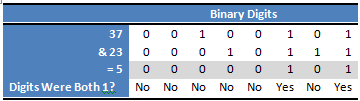Notice how each binary digit of 37 and 23 are compared, and the result has a 1 wherever both 37 and 23 had a 1, and the result has a 0 otherwise.

A common way of thinking about binary digits is as true or false. That is, 1 is equivalent to true and 0 is equivalent to false. This makes the & operator make more sense.

When we compare two booleans, we normally do boolean1 && boolean2. That expression is only true if both boolean1 and boolean2 are true. In the same way, integer1 & integer2 is equivalent, as the & operator only outputs a 1 when both binary digits of our two integers are 1.

Here's a table that represents that idea: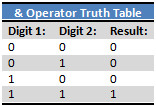A neat little use of the & operator is to check whether a number is even or odd. For integers we can simply check the rightmost bit (also called the least significant bit) to determine if the integer is odd or even. This is because when converting to base 10, the rightmost bit represents 20 or 1. When the rightmost bit is 1, we know that our number is odd since we're adding 1 to a bunch of powers of two which will always be even. When the rightmost bit is 0, we know our number will be even, since it simply consists of adding up a bunch of even numbers.

Here's an example:

 1 2 var randInt:int = int(Math.random()*1000);  3 if(randInt & 1)  4 {  5  trace("Odd number.");  6 }  7 else  8 {  9  trace("Even number.");  10 } 

On my computer, this method was about 66% faster than using randInt % 2 to check for even and odd numbers. That's quite a performance boost!

## The | Operator

Up next is the bitwise OR operator, |. As you may have guessed, the | operator is to the || operator as the & operator is to the && operator. The | operator compares each binary digit across two integers and gives back a 1 if either of them are 1. Again, this is similar to the || operator with booleans.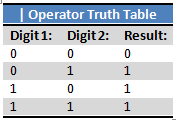Let's take a look at the same example as before, except now using the | operator instead of the & operator. We're now doing 37 | 23 which equals 55: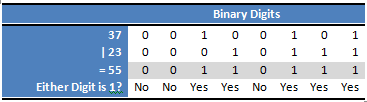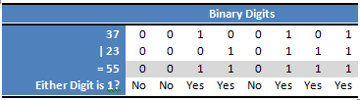### Flags: A Use of the & and | Operators

We can take advantage of the & and | operators to allow us to pass multiple options to a function in a single int.

Let's take a look at a possible situation. We're building a pop-up window class. At the bottom of it, we can have a Yes, No, Okay, or Cancel button or any combination of those - how should we do this? Here's the hard way:

 1 2 public class PopupWindow extends Sprite  3 {  4  // Variables, Constructor, etc...  5 6 7  public static void showPopup(yesButton:Boolean, noButton:Boolean, okayButton:Boolean, cancelButton:Boolean)  8  {  9  if(yesButton)  10  {  11  // add YES button  12  }  13   14  if(noButton)  15  {  16  // add NO Button  17  }  18  // and so on for the rest of the buttons  19  }  20 } 

Is this horrible? No. But it is bad, if you're a programmer, to have to look up the order of arguments every time you call the function. It's also annoying - for example, if you only want to show the Cancel button, you have to set all the other Booleans to false.

Let's use what we learned about & and | to make a better solution:

 1 2 public class PopupWindow extends Sprite  3 {  4  public static const YES:int = 1;  5  public static const NO:int = 2;  6  public static const OKAY:int = 4;  7  public static const CANCEL:int = 8;  8 9  public static void showPopup(buttons:int)  10  {  11  if(buttons & YES)  12  {  13  // add YES button  14  }  15   16  if(buttons & NO)  17  {  18  // add NO button  19  }  20  }  21 } 

How would a programmer call the function so the Yes button, No button, and Cancel button are showing? Like this:

 1 2 PopupWindow.show(PopupWindow.YES | PopupWindow.NO | PopupWindow.CANCEL); 

What's going on? It's important to note that our constants in the second example are all powers of two. So, if we look at their binary forms, we will notice they all have one digit equal to 1, and the rest equal to 0. In fact, they each have a different digit equal to 1. This means that no matter how we combine them with |, every combination will give us a unique number. Looking at it in a different way, out result of our | statement will be a binary number with a 1 wherever our options had a 1.

For our current example we have PopupWindow.YES | PopupWindow.NO | PopupWindow.CANCEL which is equivalent to 1 | 2 | 8 which rewritten in binary is 00000001 | 00000010 | 00001000 which gives us a result of 00001011.

Now, in our showPopup() function, we use & to check which options were passed in. For example, when we check buttons & YES, all the bits in YES are equal to 0 except the very rightmost one. So, we are essentially checking if the rightmost bit in buttons is a 1 or not. If it is, buttons & YES will not equal 0 and anything in the if statement will be executed. Conversely, if the rightmost bit in buttons is 0, buttons & YES will equal 0, and the if statement will not be executed.

## The ~ Operator

The bitwise NOT operator is slightly different than the two we've looked at so far. Instead of taking an integer on each side of it, it takes an integer only after it. This is just like the ! operator, and, not surprisingly, it does a similar thing. In fact, just as ! flips a boolean from true to false or vice versa, the ~ operator reverses each binary digit in an integer: from 0 to 1 and 1 to 0: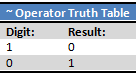A quick example. Say we have the integer 37, or 00100101. ~37 is then 11011010. What's the base 10 value of this? Well...

### Two's Complement, uint vs. int, and More!

Now the fun begins! We're going to take a closer look at binary numbers on a computer. Let's start with the uint. As mentioned before, a uint is typically 4 bytes or 32 bits long, meaning it has 32 binary digits. This is easy to understand: to get the base 10 value we simply convert the number to base 10 regularly. We'll always get a positive number.

But how about the int? It also uses 32 bits, but how does it store negative numbers? If you guessed that the first digit is used to store the sign, you're on the right path. Let's take a look at the two's complement system for storing binary numbers. While we won't go into all the details here, a two's complement system is used because it makes binary arithmetic easy.

To find the two's complement of a binary number, we simply flip all the bits (i.e. do what the ~ operator does) and add one to the result. Let's try this out once: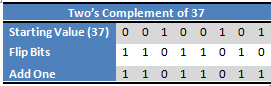We then define our result as the value -37. Why do this complicated process and not just flip the very first bit and call that -37?

Well, let's take a simple expression 37 + -37. We all know this should equal 0, and when we add the 37 to its two's complement, that's what we get: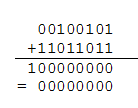Notice that since our integers only hold eight binary digits, the 1 in our result is dropped, and we end up with 0, as we should.

To recap, to find the negative of a number, we simply take its two's complement. We can do this by inverting all the bits and adding one.

Want to try this yourself? Add trace(~37+1); to an AS3 file, then compile and run it. You'll see -37 is printed, as it should be.

There is also a little shortcut to do this by hand: starting from the right, work to the left until you reach a 1. Flip all the bits to the left of this first 1.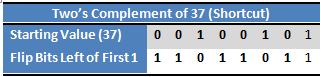When we're looking at a signed binary number (in other words, one that can be negative, an int not a uint), we can look at the leftmost digit to tell whether it's negative or positive. If it's a 0, then the number is positive and we can convert to base 10 simply by calculating its base 10 value. If the leftmost bit is a 1, then the number is negative, so we take the two's complement of the number to get its positive value and then simply add a negative sign.

For example, if we have 11110010, we know it is a negative number. We can find it's two's complement by flipping all the digits to the left of the rightmost 1, giving us 00001110. This equals 13, so we know 11110010 equals -13.

## The ^ Operator

We're back to the bitwise operators, and up next is the bitwise XOR operator. There is no equivalent boolean operator to this one.

The ^ operator is similar to the & and | operators in that it takes an int or uint on both sides. When it is calculating the resulting number, it again compares the binary digits of these numbers. If one or the other is a 1, it will insert a 1 in to the result, otherwise it will insert a 0. This is where the name XOR, or "exclusive or" comes from.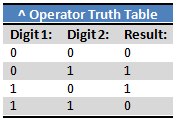Let's take a look at our usual example: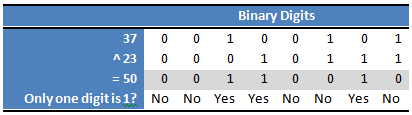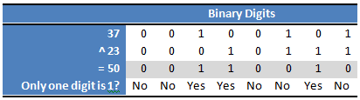The ^ operator does have uses - it's especially good for toggling binary digits - but we won't cover any practical applications in this article.

## The << Operator

We're now on the bitshift operators, specifically the bitwise left shift operator here.

These work a little differently than before. Instead of comparing two integers like &, |, and ^ did, these operators shift an integer. On the left side of the operator is the integer that is being shifted, and on the right is how much to shift by. So, for example, 37 << 3 is shifting the number 37 to the left by 3 places. Of course, we're working with the binary representation of 37.

Let's take a look at this example (remember, we're just going to pretend integers only have 8 bits instead of 32). Here we have the number 37 sitting on its nice block of memory 8 bits wide.Alright, let's slide all the digits over to the left by 3, as  37 << 3 would do: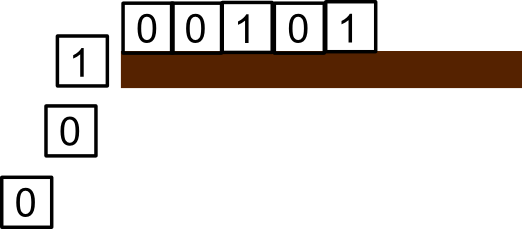But now we have a small problem - what do we do with the 3 open bits of memory where we moved the digits from?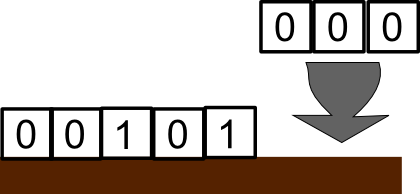Of course! Any empty spots are just replaced with 0s. We end up with 00101000 . And that's all there is to the left bitshift. Keep in mind that Flash always thinks the result of a left bitshift is an int, not a uint. So if you need a uint for some reason, you'll have to cast it to a uint like this: uint(37 << 3). This casting doesn't actually change any of the binary information, just how Flash interprets it (the whole two's complement thing).

An interesting feature of the left bitshift is that it is the same as multiplying a number by two to the shiftAmount-th power. So, 37 << 3 == 37 * Math.pow(2,3) == 37 * 8. If you can use the left shift instead of Math.pow, you'll see a huge performance increase.

You may have noticed that the binary number we ended up with did not equal 37 * 8. This is just from our use of only 8 bits of memory for integers; if you try it in ActionScript, you'll get the correct result. Or, try it with the demo at the top of the page!

## The >> Operator

Now that we understand the left bitshift, the next one, the right bitshift, will be easy. Everything slides to the right the amount we specify. The only slight difference is what the empty bits get filled with.

If we're starting with a negative number (a binary number where the leftmost bit is a 1), all the empty spaces are filled with a 1. If we're starting with a positive number (where the leftmost bit, or most significant bit, is a 0), then all the empty spaces are filled with a 0. Again, this all goes back to two's complement.

While this sounds complicated, it basically just preserves the sign of the number we start with. So  -8 >> 2 == -2 while 8 >> 2 == 2. I'd recommend trying those out on paper yourself.

Since >> is the opposite of <<, it's not surprising that shifting a number to the right is the same as dividing it by 2 to the power of shiftAmount. You may have noticed this from the example above. Again, if you can use this to avoid calling Math.pow, you'll get a significant performance boost.

## The >>> Operator

Our final bitwise operator is the bitwise unsigned right shift. This is very similar to the regular bitwise right shift, except that all empty bits on the left are filled with 0s. This means the result of this operator is always a positive integer and it always treats the integer being shifted as an unsigned integer. We won't run through an example of this in this section, but we'll see a use for it very shortly.

## Using Bitwise Operators to Work With Colors

One of the most practical uses of bitwise operators in Actionscript 3 is working with colors, which are stored typically as uints.

The standard format for colors is to write them in hexadecimal: 0xAARRGGBB - each letter represents a hexadecimal digit. Here, the first two hexadecimal digits, which are equivalent to the first eight binary digits, represent our alpha, or transparency. The next eight bits represent the amount of red in our color (so an integer from 0 to 255), the next eight the amount of green, and the final eight represent the amount of blue in our color.

Without bitwise operators, it's extremely difficult to work with colors in this format - but with them it's easy!

Challenge 1: Find the amount of blue in a color: Using the & operator, try to find the amount of blue in an arbitrary color.

 1 2 public function findBlueComponent(color:uint):uint  3 {  4  // Your code here!  5 } 

We need a way to 'erase' or mask all the other data in color and just have the blue component left. This is easy, actually! If we take color & 0x000000FF - or, written more simply, color & 0xFF - we end up with only the blue component.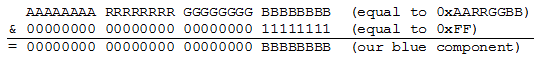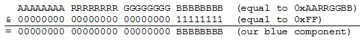As you can see from above and you learned in the description of the & operator, any binary digit & 0 will always equal 0, while any binary digit & 1 will keep its value. So if we mask our color by 0xFF which only has 1s where the blue component of our color is located, we end up with just the blue component.

Challenge 2: Find the amount of red in a color: Using two bitwise operators, try to find the amount of red in an arbitrary color.

 1 2 public function findRedComponent(color:uint):uint  3 {  4  // Your code here!  5 } 

We actually have two solutions to this problem. One would be return (color & 0xFF0000) >> 16; and the other would be  return (color >> 16) & 0xFF;

This is very similar to Challenge 1, except that we have to shift our answer at some point.

Challenge 3: Find the transparency of a color: Using only one bitwise operator, try to find the alpha of a color (an integer from 0 to 255).

 1 2 public function findAlphaComponent(color:uint):uint  3 {  4  // Your code here!  5 } 

This one is a touch trickier. We have to be careful with which right shift operater we choose. Because we're working with the leftmost digits of a uint, we want to use >>> operator. So, our answer simply is return color >>> 24;.

Final Challenge: Create a color from its components: Using the << and | operators, take the components of a color and merge them in to one uint.

 1 2 public function createColor(a:uint, r:uint, g:uint, b:uint):uint  3 {  4  // Your code here!  5 } 

Here, we have to shift each component to its correct position, and then merge them. We want Flash to treat it as an unsigned integer, so we cast it to a uint:  return uint((a << 24) | (r << 16) | (g << 8) | b);

## Compound Operators

You may have noticed I have neglected to explain the compound bitwise operators. Imagine we have an integer x. Then, x = x & 0xFF is the same as x &= 0xFF, x = x | 256 is the same as x |= 256, and so on for the rest of the compound operators.

## Conclusion

Thanks for reading this article! I hope you now understand bitwise operators and can utilize them in your AS3 code (or in many other languages!). As always, if you have any questions or comments, please leave them below.